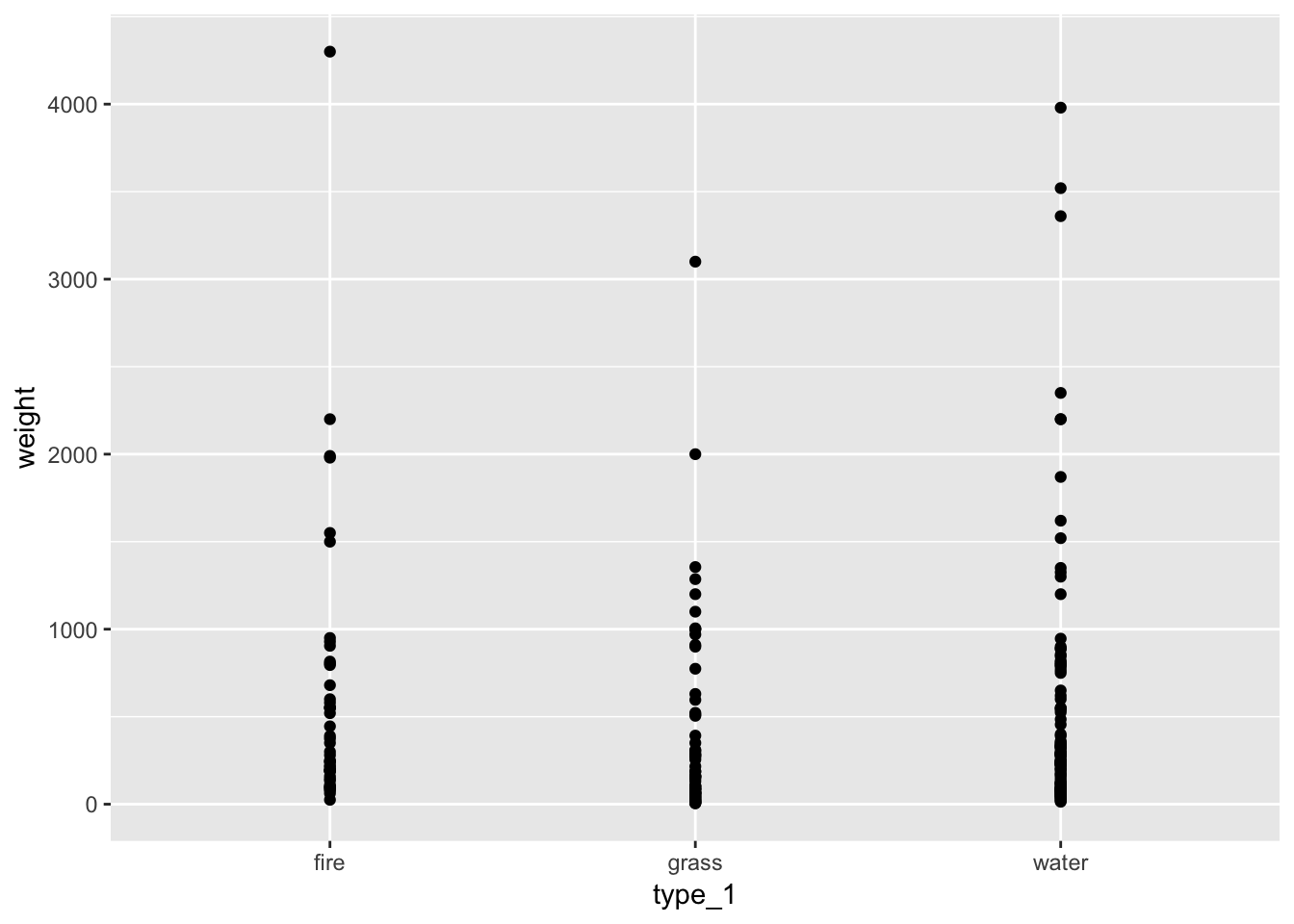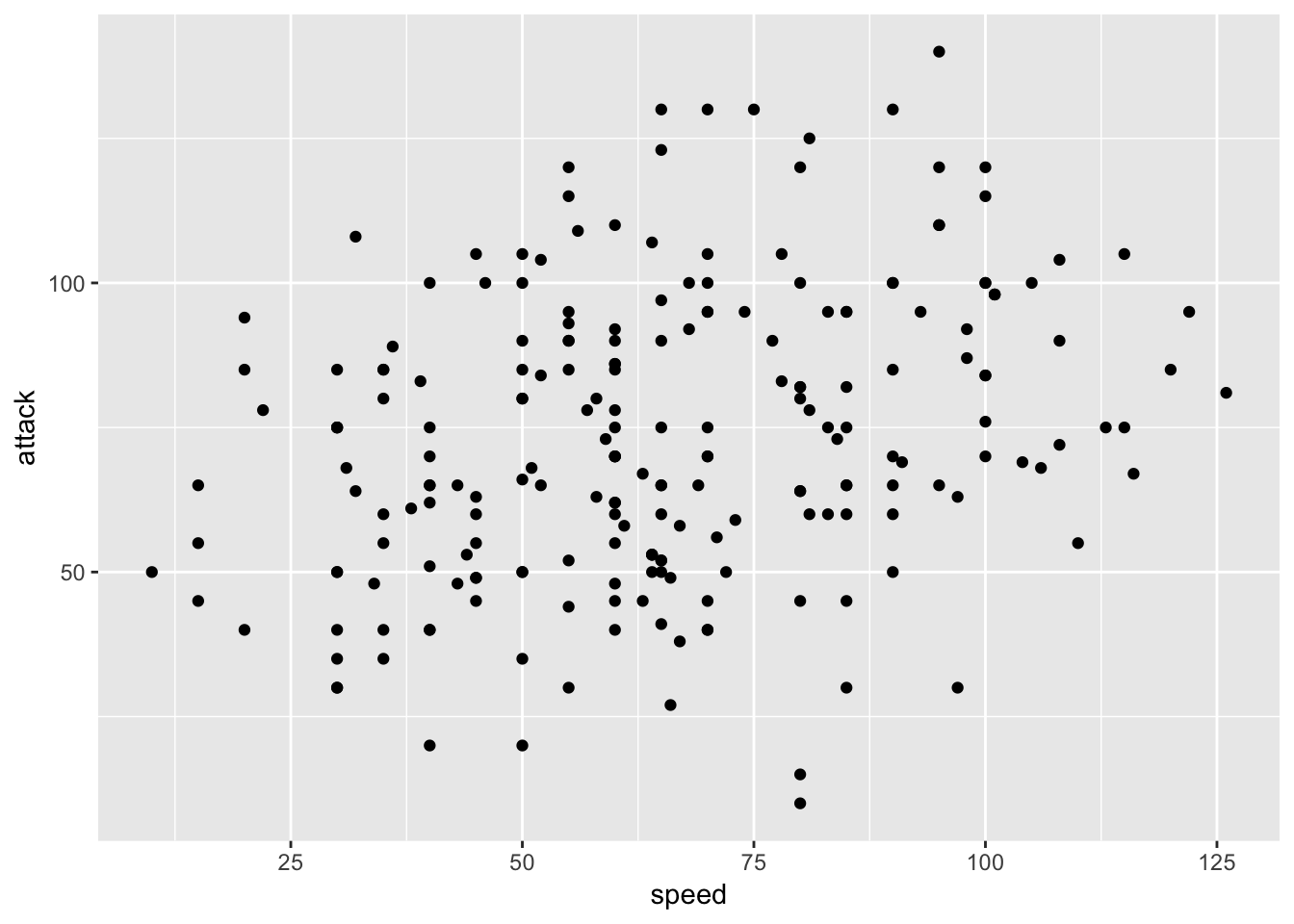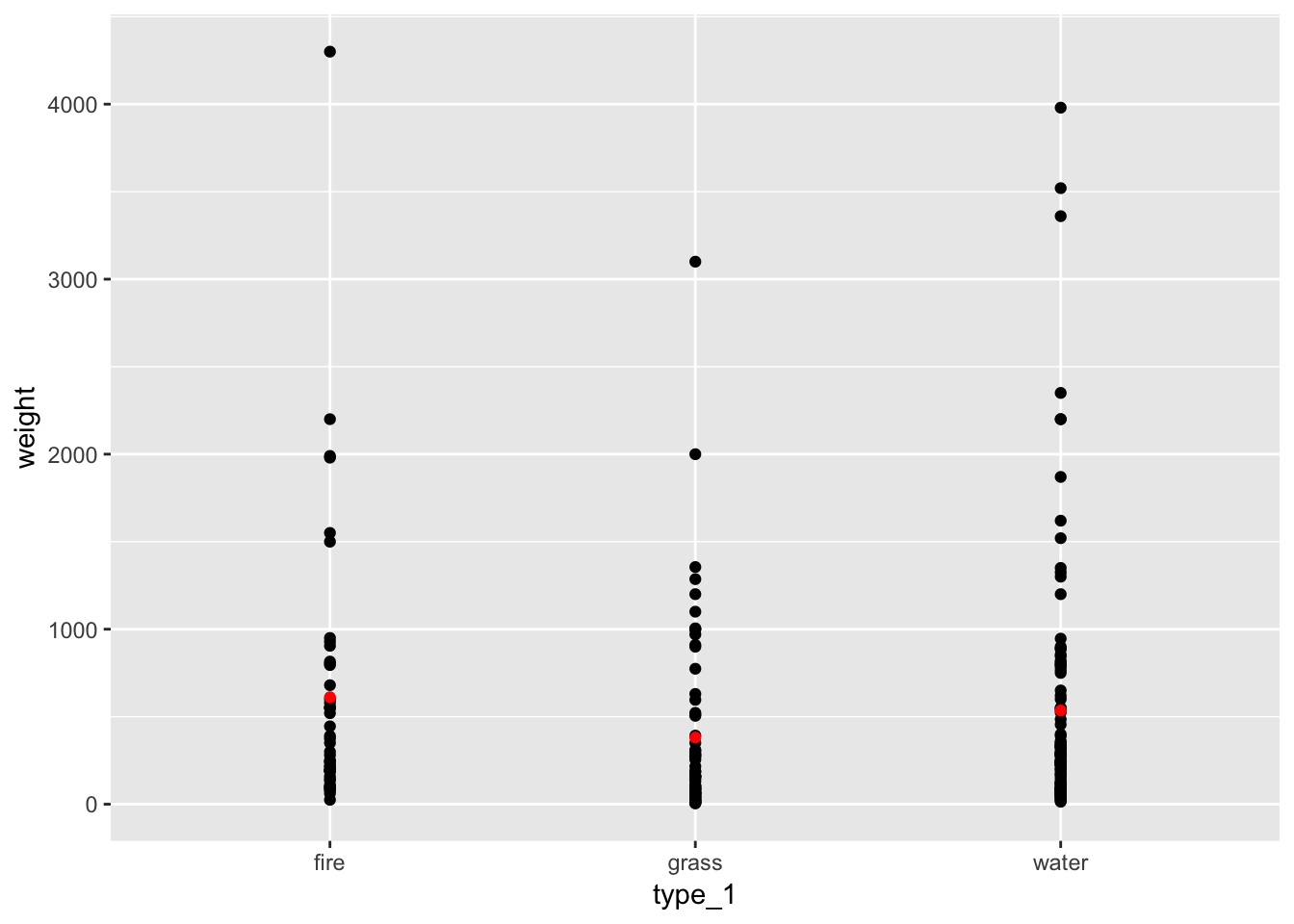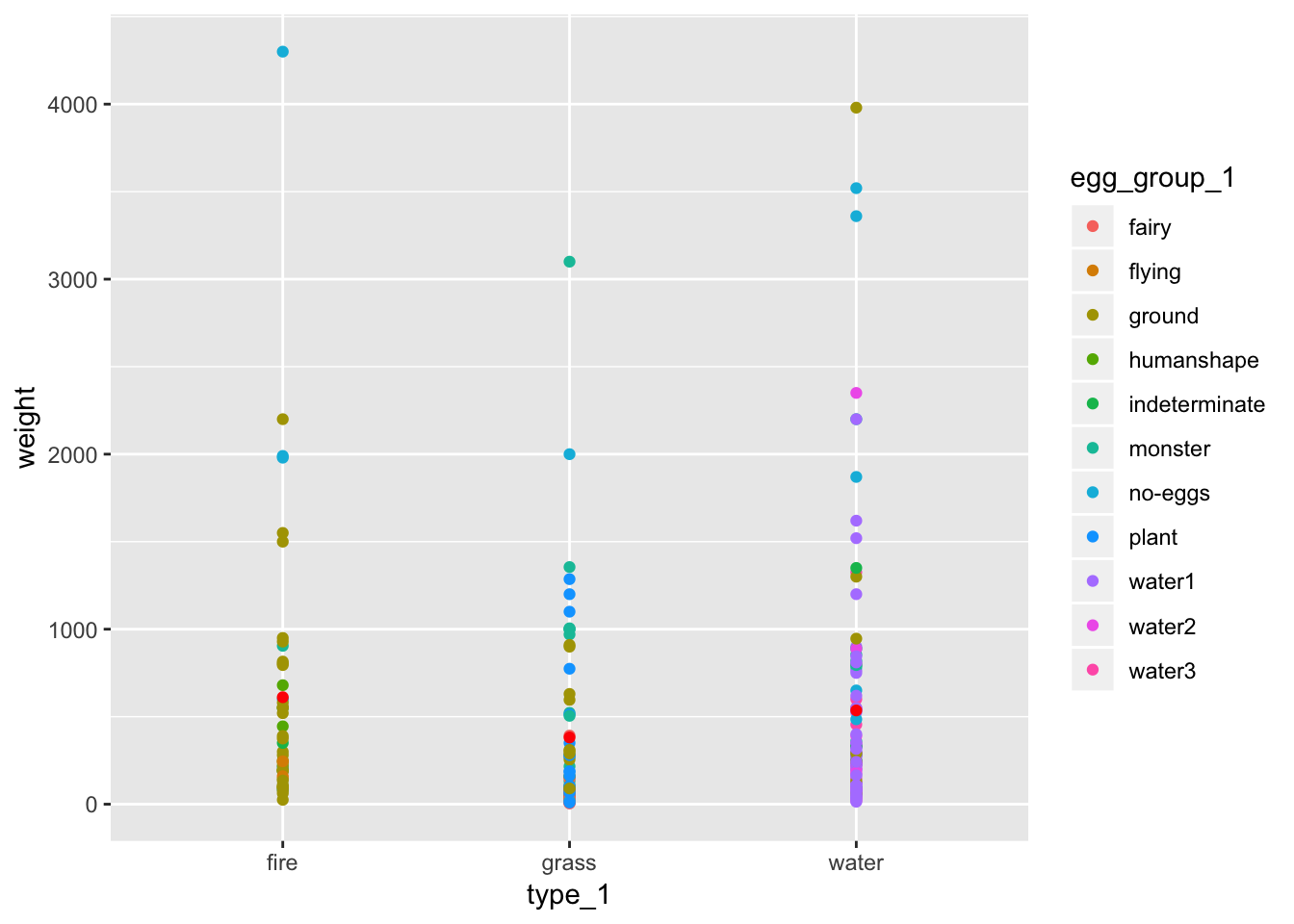Writing efficient and streamlined R code with help from the new rlang package

The powerful new rlang package developed by Lionel Henry and Hadley Wickham at RStudio gives R programmers new tools to control evaluation of variables, compose formulas and functions and modify environments. R programmers can tackle these tasks without rlang, but the package gives us more straightforward approaches and solutions.

Although broadly useful, to a certain extent rlang provides tools for tool builders rather than tools for casual users. In rlang, for example, you might use !! or even !!! to perform tasks. These are clearly not as easily understood out of the box as R functions with clear names such as filter() and arrange().

But what rlang adds in opaque syntax the package makes up for in powerful and concise tools. Of particular value, are rlang's tools for evaluating quoted and un-quoted variables in different contexts. For example, base R often makes use of strings as variable names as in dat[,"variable1"]. More recent tools, like those in the dplyr package, tend to use bare variable names such as select(dat, variable1). As you write your own functions rlang helps you make use of both strategies and to move between them — evaluating strings within functions that use bare-name syntax and vice-versa.

Popular R packages like dplyr and ggplot2 now use rlang to power the bare name syntax found in key functions such as select(), mutate() or aes() and rlang easily allows you to use the same strategies in your own R code.

Using ggplot2 to demonstrate the power of rlang

To illustrate the power of rlang we will build a custom plotting function with the brand new version of ggplot2 (version 3.0.0). The function will take a data frame and two column names and plot the two variables as a dot chart. In the final step we will bring in the dplyr package to help us include means on the plot.

In each example we are going to use a slice of the pokemon data set from the highcharter package.

library(dplyr)
# install.packages("highcharter")
data(pokemon, package = "highcharter")

# limit our data to water, grass, and fire types
pokemini <- pokemon[pokemon\$type_1 %in% c("water", "grass", "fire"), ]
glimpse(pokemini)
## Observations: 217
## Variables: 20
## \$ id              <dbl> 1, 2, 3, 4, 5, 6, 7, 8, 9, 37, 38, 43, 44, 45,...
## \$ pokemon         <chr> "bulbasaur", "ivysaur", "venusaur", "charmande...
## \$ species_id      <int> 1, 2, 3, 4, 5, 6, 7, 8, 9, 37, 38, 43, 44, 45,...
## \$ height          <int> 7, 10, 20, 6, 11, 17, 5, 10, 16, 6, 11, 5, 8, ...
## \$ weight          <int> 69, 130, 1000, 85, 190, 905, 90, 225, 855, 99,...
## \$ base_experience <int> 64, 142, 236, 62, 142, 240, 63, 142, 239, 60, ...
## \$ type_1          <chr> "grass", "grass", "grass", "fire", "fire", "fi...
## \$ type_2          <chr> "poison", "poison", "poison", NA, NA, "flying"...
## \$ attack          <int> 49, 62, 82, 52, 64, 84, 48, 63, 83, 41, 76, 50...
## \$ defense         <int> 49, 63, 83, 43, 58, 78, 65, 80, 100, 40, 75, 5...
## \$ hp              <int> 45, 60, 80, 39, 58, 78, 44, 59, 79, 38, 73, 45...
## \$ special_attack  <int> 65, 80, 100, 60, 80, 109, 50, 65, 85, 50, 81, ...
## \$ special_defense <int> 65, 80, 100, 50, 65, 85, 64, 80, 105, 65, 100,...
## \$ speed           <int> 45, 60, 80, 65, 80, 100, 43, 58, 78, 65, 100, ...
## \$ color_1         <chr> "#78C850", "#78C850", "#78C850", "#F08030", "#...
## \$ color_2         <chr> "#A040A0", "#A040A0", "#A040A0", NA, NA, "#A89...
## \$ color_f         <chr> "#81A763", "#81A763", "#81A763", "#F08030", "#...
## \$ egg_group_1     <chr> "monster", "monster", "monster", "monster", "m...
## \$ egg_group_2     <chr> "plant", "plant", "plant", "dragon", "dragon",...
## \$ url_image       <chr> "1.png", "2.png", "3.png", "4.png", "5.png", "...

A first go

Create a plot_points1() function and try calling the function with bare name arguments.

library(ggplot2)

plot_points1 <- function(.data, x, y) {
ggplot(.data, aes(x, y)) +
geom_point()
}

# Feed the function bare variable names
plot_points1(pokemini, type_1, weight)
## Error in FUN(X[[i]], ...): object 'type_1' not found

But you’ll notice that running the example gives an error. The name we provided as argument one, type_1, is evaluated prematurely in the wrong context. In the past we might have used aes_string() instead of aes() and asked the user to provide string inputs (i.e. plot_points1(pokemini, "type_1", "weight"). But this is inconsistent with ggplot2 behavior and aes_string() is now soft-deprecated in favor of the following strategy. We want our plotting function to work without aes_string() and with bare names.

It lives!

To alter plot_points1() to allow bare name inputs we will use two new tools provided by rlang, enquo() and !! (referred to as “bang bang”). enquo() is a means of preserving an argument for later evaluation. The !! operator is the compliment and allows us to evaluate a captured value.

plot_points2 <- function(.data, x, y) {
x <- enquo(x)
y <- enquo(y)
ggplot(.data, aes(!!x, !!y)) +
geom_point()
}
plot_points2(pokemini, type_1, weight)Cool! But, let’s take a moment to break down what contributed to our success.

x <- enquo(x)
y <- enquo(y)

Our function begins by capturing the arguments x and y. This is critical. Think about handing a Lego set to a friend. Instinctively they use the default instructions to build the set. What if instead we handed them a Lego set and a different booklet of instructions? Now they can build an entirely different creation with the same Legos. That is the power of enquo(). Before calling x <- enquo(x) R had its own plans for evaluating x, but now we have control over the instructions for evaluating x. In our case, we need to evaluate the arguments x and y inside aes().

aes(!!x, !!y)

In our call to aes() we can force evaluation of the captured x and y using !!. Jumping back to Legos, this is us telling our friend to build using the new instructions. Within the proper context, the data passed to ggplot(), our values stored in x and y, type_1 and weight respectively, are understood, no more error.

For good measure let’s try another combination of variables.

plot_points2(pokemini, speed, attack)Calculating and plotting averages

Let’s add yet another feature to our plot_points() function. We will include mean values in our plot. Fortunately for us dplyr’s verbs support !!, so with our new knowledge of !! and enquo() we can compute averages on the fly. Take a look at the final product followed by a breakdown of the code.

plot_points3 <- function(.data, x, y) {
x <- enquo(x)
y <- enquo(y)
averages <- .data %>%
group_by(!!x) %>%
summarise(avg_y = mean(!!y, na.rm = TRUE))

ggplot() +
geom_point(data = .data, aes(!!x, !!y)) +
geom_point(data = averages, aes(!!x, avg_y), color = "red")
}

Our new function computes averages for our y variable and adds an additional set of points to our plot. Let’s take a look at the new chunk of code,

averages <- .data %>%
group_by(!!x) %>%
summarise(avg_y = mean(!!y, na.rm = TRUE))

In this snippet we limit our data to the two relevant variables, group the data by our x variable, and compute mean values for each group of y values.

group_by(!!x)

As in aes() and select() we use !! to evaluate x. Remember, we captured x with enquo(). At this point our data is grouped by type_1.

summarise(avg_y = mean(!!y, na.rm = TRUE))

In our final step we evaluate y, !!y, and pass the column to mean(). With that we have calculated our mean values. Let’s try out the function.

plot_points3(pokemini, type_1, weight)A final transmogrification

As our first walk through rlang concludes, let’s look at one last version of the plot_points() function. In this version any number of additional named arguments are passed on to aes(). This, for example, would allow us to color points by another variable egg_group_1. This is done with the help of the ellipsis, ..., which allows a function to accept any number of additional arguments.

plot_points4 <- function(.data, x, y, ...) {
x <- enquo(x)
y <- enquo(y)
args <- enquos(...)

averages <- .data %>%
group_by(!!x) %>%
summarise(avg_y = mean(!!y, na.rm = TRUE))

ggplot(.data, aes(!!x, !!y, !!!args)) +
geom_point() +
geom_point(mapping = aes(!!x, avg_y), data = averages,color = "red")
}
plot_points4(pokemini, type_1, weight, color = egg_group_1)To learn more about enquo(), !!, and friends checkout the rlang package, the dplyr vignette on programming with dplyr, and upcoming posts right here!

Posted in R

One response

1.John Mount says:

Neat. I suggest readers also give our wrapr-based solution a try:

library("ggplot2")
library("dplyr")
library("wrapr")
data(pokemon, package = "highcharter")
pokemini <- pokemon[pokemon\$type_1 %in% qc(water, grass, fire), , drop = FALSE]

plot_pointsw <- function(.data, x, y, color) c(
X = x,
Y = y,
COLOR = color
) %in_block% {
averages <- .data %>%
group_by(X) %>%
summarise(avg_y = mean(Y, na.rm = TRUE))

ggplot(.data, aes(X, Y, color = COLOR)) +
geom_point() +
geom_point(mapping = aes(X, avg_y), data = averages, color = "red")
}

plot_pointsw(pokemini, "type_1", "weight", "egg_group_1")